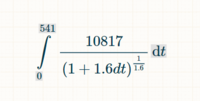# Integral Problem

#### nate12o6

##### New member
Hi everyone,

Its been about 12 years sense i took my calc courses but am running into a problem that I am trying to figure out.

If I have the value of an integral is there any way to solve for a variable that makes up the integral.

Here is an example:

My integral (Not sure how to format this) is from 0 -> 541 f(t)dt =10817/((1+((1.6)(d)(t)))^(1/1.6) which equals 370,367. I am trying to solve for d.

I have attached a picture that formats the formula i bit better.Again. Trying to solve for d when i know the integral is equal to 370,367.

Thanks for any help!

#### MarkFL

##### Super Moderator
Staff member
Hello, and welcome to FMH!I"m going to change $$d$$ to $$a$$ to avoid confusion and write:

$$\displaystyle 10817\int_0^{541} \frac{1}{\left(1+\frac{8}{5}at\right)^{\frac{5}{8}}}\,dt=370367$$

$$\displaystyle \int_0^{541} \frac{1}{\left(1+\frac{8}{5}at\right)^{\frac{5}{8}}}\,dt=\frac{370367}{10817}$$

Let:

$$\displaystyle u=1+\frac{8}{5}at\implies du=\frac{8}{5}dt$$

And our definite integral becomes:

$$\displaystyle \int_1^{1+\frac{4328}{5}a} u^{-\frac{5}{8}}\,du=\frac{2962936}{54085}$$

Can you proceed?